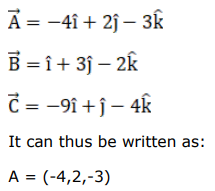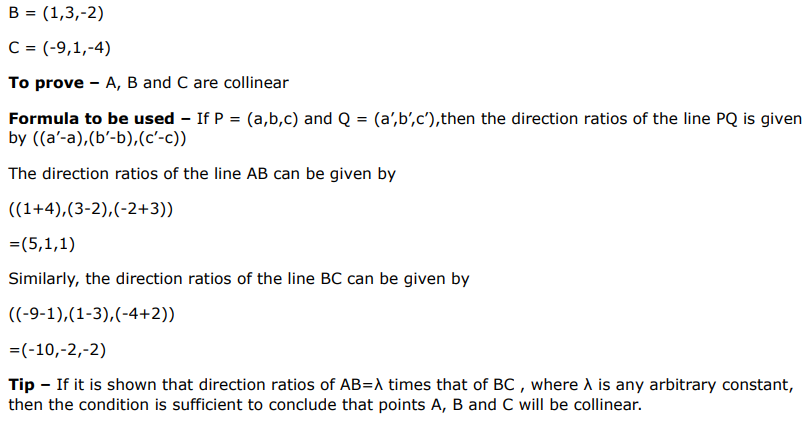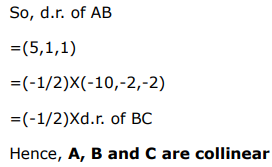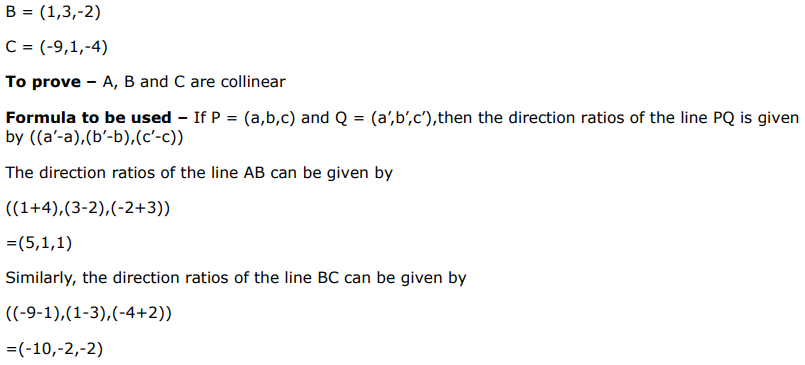# The position vectors of three points A, B and C are

Question:

The position vectors of three points $A, B$ and $C$ are $(-4 \hat{\mathrm{i}}+2 \hat{\mathrm{j}}-3 \hat{\mathrm{k}}),(\hat{\mathrm{i}}+3 \hat{\mathrm{j}}-2 \hat{\mathrm{k}})$ and $(-9 \hat{i}+\hat{j}-4 \hat{k})$ respectively. show that the points $A, B$ and $C$ are collinear.

Solution: